# NCERT Solutions for Class 6 Maths Chapter 4 – Basic Geometrical Ideas

Download NCERT Solutions for Class 6 Maths Chapter 4 – Basic Geometrical Ideas

###NCERT Solutions for Class 6 Maths Chapter 4 – Basic Geometrical Ideas comprises of the 6 Exercises

NCERT Solutions for Class 6 Maths Chapter 4 Exercise 4.1 Video Explanation

NCERT Solutions for Class 6 Maths Chapter 4 Exercise 4.2 Video Explanation

NCERT Solutions for Class 6 Maths Chapter 4 Exercise 4.3 Video Explanation

NCERT Solutions for Class 6 Maths Chapter 4 Exercise 4.4 Video Explanation

NCERT Solutions for Class 6 Maths Chapter 4 Exercise 4.5 Video Explanation

NCERT Solutions for Class 6 Maths Chapter 4 Exercise 4.6 Video Explanation

### NCERT Solutions for Class 6 Maths Chapter 4 Exercise 4.1

Ques.1. Use the figure to name: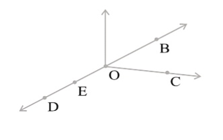(a) Five points
(b) A line
(c) Four rays
(d) Five line segments

Sol.1.

a) Five points are O, B, D, C, E
b) A line$\overleftrightarrow { DB }$
c) Four rays are$\overrightarrow { OD } ,\overrightarrow { ED } ,\overrightarrow { OC } ,\overrightarrow { OB }$
d) Five line segments are$\overline { DE } ,\overline { OE } ,\overline { OC } ,\overline { OB } ,\overline { OD }$

Ques.2. Name the line given in all possible (twelve) ways, choosing only two letters at a time from the four given.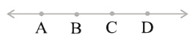Sol. 2.$\overline { AB }, \overline { AC}, \overline { AD }, \overline { BC }, \overline { BD }, \overline { CD }, \overline { BA }, \overline { CA }, \overline { DA }, \overline { CB }, \overline { DB }, \overline { DC }$

Ques.3. Use the figure to name: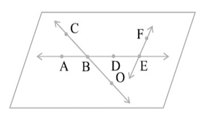(a) Line containing point E.
(b) Line passing through A.
(c) Line on which O lies
(d) Two pairs of intersecting lines.

Sol. 3.

a) Line containing point E is$\overline { AE }$
b) Line passing through A is$\overline { AE }$
c) Line on which O lies is$\overline { OC }$
d) Two pairs of intersecting lines are$\overline { AD }, \overline { CO }$ and$\overline { AE }, \overline { FE }$

Ques.4. How many lines can pass through
(a) one given point?
(b) two given points?

Sol. 4.
a) Infinite lines can pass through a given point.
b) Only one line can pass through two given points.

Ques.5.Draw a rough figure and label suitably in each of the following cases:

(a) Point P lies on$\overline { AB }$.
(b)$\overline { XY }$ and$\overline { PQ }$ intersect at M.
(c) Line l contains E and F but not D.
(d)$\overline { OP }$ and$\overline { OQ }$ meet at O.

Sol. 5. a)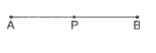b)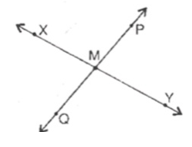c)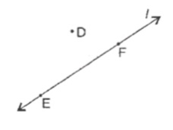d)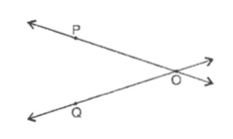Ques.6. Consider the following figure of line$\overline { MN }$. Say whether following statements are true or false in context of the given figure.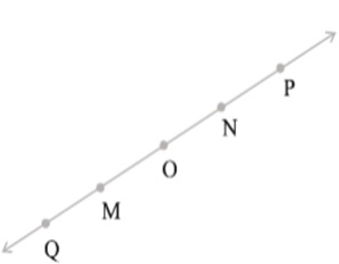(a) Q, M, O, N, P are points on the line$\overline { MN }$.
(b) M, O, N are points on a line segment$\overline { MN }$ .
(c) M and N are end points of line segment$\overline { MN }$ .
(d) O and N are end points of line segment$\overline { OP }$.
(e) M is one of the end points of line segment$\overline { QO }$ .
(f) M is point on ray$\overline { OP }$.
(g) Ray$\overline { OP }$ is different from ray$\overline { QP }$.
(h) Ray$\overline { OP }$ is same as ray$\overline { OM }$.
(i) Ray$\overline { OM }$, is not opposite to ray$\overline { OP }$.
(j) O is not an initial point of$\overline { OP }$.
(k) N is the initial point of$\overline { NP }$ and$\overline { NM }$.

Sol. 6.

(a) True, line$\overline { MN }$ has the two ends extended from point M and N. Hence all the point in this line in the given figure will exist on the line.
(b) True, since all the three points lies within this line.
(c) True, since M may be seen as starting point and N as end point or vise versa of the given line segment.
(d) False, because N lies somewhere in the middle of the line segment$\overline { OP }$.
(e) False, because M lies somewhere in the middle of the line segment$\overline { QO }$.
(f) False, because M lies before the starting point of the ray$\overline { OP }$. Hence M cannot lie on the ray$\overline { OP }$.
(g) True, because for two rays to be same they must have same starting point.
(h) False, because the point M and P lie to the opposite side of O. So they can not be same ray.
(i) False, because the point M and P lie to the opposite side of O. So they are opposite to each other.
(j) False, O is initial point of$\overline { OP }$.
(k) True, because both line originates from common point N.

### NCERT Solutions for Class 6 Maths Chapter 4 Exercise 4.2

Ques. 1. Classify the following curves as (i) Open or (ii) Closed.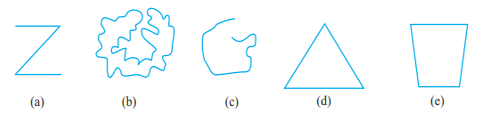Sol. 1. (a) Open
(b) Closed
(c) Open
(d) Closed
(e) Closed

Ques. 2. Draw rough diagrams to illustrate the following:

(a) Open curve
(b) Closed curve.

Sol. 2.

(a) Open curve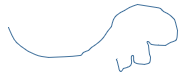(b) Closed curveQues. 3. Draw any polygon and shade its interior.

Sol. 3.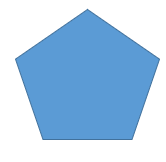It is a polygon with 5 sides, known as pentagon.

Ques. 4. Consider the given figure and answer the questions:(a) Is it a curve?
(b) Is it closed?

Sol. 4.   (a) Yes it is a curve.
(b) Yes it is closed as it forms a closed boundary.

Ques. 5. Illustrate, if possible, each one of the following with a rough diagram:
(a) A closed curve that is not a polygon.
(b) An open curve made up entirely of line segments.
(c) A polygon with two sides.

Sol. 5.    (a) A closed curve that is not a polygon.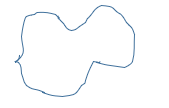(b) An open curve made up entirely of line segments.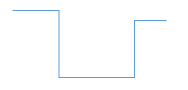(c) A polygon with two sides- Not possible, because a polygon is a closed figure made up of line segments. It requires, minimum 3 line segments to form a polygon, i.e., a triangle.

### NCERT Solutions for Class 6 Maths Chapter 4 Exercise 4.3

Ques. 1. Name the angles in the given figure.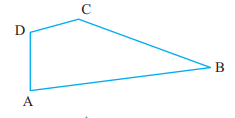Sol. 1.    Angles in the given figure are
∠B or ∠ABC or ∠CBA,
∠C or ∠BCD or ∠DCB,

Ques. 2. In the given diagram, name the point(s)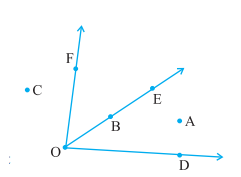(a) In the interior of ∠DOE
(b) In the exterior of ∠EOF
(c) On ∠EOF

Sol. 2.    (a) A is the point in the interior of ∠DOE.
(b) A, C, D are the points that lie in the exterior of ∠EOF.
(c) E, B, O, F are the points that lie on ∠EOF.

Ques. 3. Draw rough diagrams of two angles such that they have

(a) One point in common.
(b) Two points in common.
(c) Three points in common.
(d) Four points in common.
(e) One ray in common.

Sol. 3.    (a) One point in common.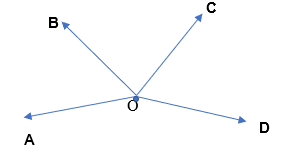(b) Two points in common.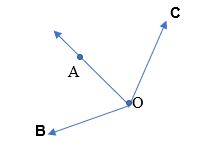(c) Three points in common.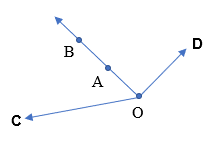(d) Four points in common.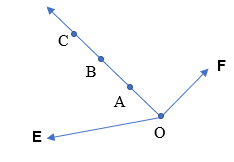(e) One ray in common.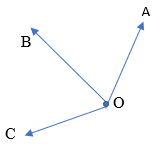### NCERT Solutions for Class 6 Maths Chapter 4 Exercise 4.4

Ques. 1. Draw a rough sketch of a triangle ABC. Mark a point P in its interior and a point Q in its exterior. Is the point A in its exterior or in its interior?

Sol. 1.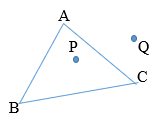Point A lies on the triangle. It is neither in its exterior nor in its interior. It is a vertex.

Ques. 2. (a) Identify three triangles in the figure.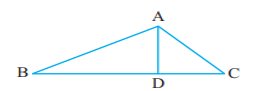(b) Write the names of seven angles.
(c) Write the names of six line segments.
(d) Which two triangles have ∠B as common?

Sol. 2.    (a) The three triangles in the figure are ∆ABD, ∆ADC and ∆ABC.
(b) The seven angles are ∠ABC or ∠B, ∠ACD or ∠C, ∠ADC, ∠ADB, ∠BAD, ∠DAC, ∠BAC or ∠A.
(c) The six line segments are AB, BD, DC, BC, AC and AD.
(d) ∆ABC and ∆ABD have ∠B in common.

### NCERT Solutions for Class 6 Maths Chapter 4 Exercise 4.5

Ques. 1. Draw a rough sketch of a quadrilateral PQRS. Draw its diagonals. Name them. Is the meeting point of the diagonals in the interior or exterior of the quadrilateral?

Sol. 1.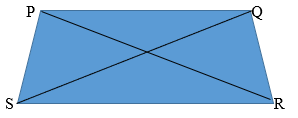The diagonals are PR and QS. They meet in the interior of the quadrilateral PQRS.

Ques. 2. Draw a rough sketch of a quadrilateral KLMN. State,
(a) two pairs of opposite sides,
(b) two pairs of opposite angles,
(c) two pairs of adjacent sides,
(d) two pairs of adjacent angles.

Sol. 2.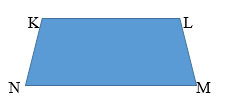(a) two pairs of opposite sides are (KL, NM) and (KN, LM).
(b) two pairs of opposite angles are (∠K, ∠M) and (∠L, ∠N).
(c) two pairs of adjacent sides are (KL, LM) and (KN, NM).
(d) two pairs of adjacent angles are (∠K, ∠L) and (∠N, ∠M).

### NCERT Solutions for Class 6 Maths Chapter 4 Exercise 4.6

Ques. 1. From the figure, identify: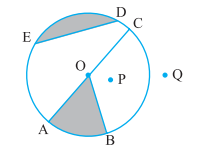(a) the centre of circle
(c) a diameter
(d) a chord
(e) two points in the interior
(f) a point in the exterior
(g) a sector
(h) a segment

Sol. 1.   (a) O is the centre of circle.
(b) OC, OA and OB are the three radii.
(c) AC is the diameter.
(d) ED is the chord.
(e) O and P are the two points in the interior of the circle.
(f) Q is a point in the exterior.
(g) OAB is a sector (shaded portion)
(h) ED is a segment (shaded portion)

Ques. 2. (a) Is every diameter of a circle also a chord?
(b) Is every chord of a circle also a diameter?

Sol.  2.   (a) Yes,  every diameter of a circle is a chord, since it cuts the circle into 2 segments.
(b) No, every chord of a circle is not necessarily a diameter, because it might not pass through the centre of the circle.

Ques. 3. Draw any circle and mark

(a) its centre
(c) a diameter
(d) a sector
(e) a segment
(f) a point in its interior
(g) a point in its exterior
(h) an arc

Sol. 3.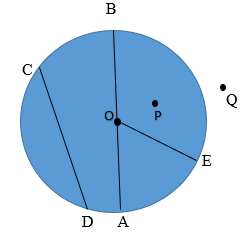(a) O is the centre.
(b) OA, OB, OE are the radii.
(c) AB is the diameter.
(d) AOE is a sector.
(e) CD is a segment.
(f) P, O are the points in the interior of the circle.
(g) Q is a point in the exterior of the circle.
(h) AE is an arc.

Ques. 4. Say true or false:
(a) Two diameters of a circle will necessarily intersect.
(b) The centre of a circle is always in its interior.

Sol. 4.   (a) True. The diameters always pass through the centre of the circle. Hence, they will necessarily intersect at the centre.
(b) True. The centre of a circle lies within the circle.

####NCERT Solutions Class 6

This Chapter contains the Exercises relating to the following topics , which are discussed in Chapter 4 – Basic Geometrical Ideas Class 6 NCERT book  : –

• Exercise 4.1 Introduction
• Exercise 4.2 Points
• Exercise 4.3 A Line Segment
• Exercise 4.4 A Line
• Exercise 4.5 Intersecting Lines
• Exercise 4.6 Parallel Lines
• Exercise 4.7 Ray
• Exercise 4.8 Curves
• Exercise 4.9 Polygons
• Exercise 4.10 Angles
• Exercise 4.11 Triangles